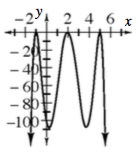### Home > INT3 > Chapter 10 > Lesson 10.1.4 > Problem10-59

10-59.

Sketch a graph of each of the following functions. Describe the graphs.

1. $y = \left(x + 1\right)^{2}\left(x - 2\right)^{2}\left(x - 5\right)^{2}$

Is this graph even or odd?

To solve for the $x$-values, set the equation to zero.
Will the line bounce off or go through these points?

1. $y = -\left(x + 1\right)^{2}\left(x - 2\right)^{2}\left(x - 5\right)^{2}$

Follow the steps from part (a). What similarities do you notice between the two graphs?